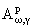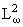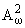A.M. Jerbashian

On Functions of w-bounded Type in the Half-plane

This paper gives the basic representations of the general theory of functions of w-bounded type in the upper half-plane. The starting point are the canonical representations of some Banach spacesof holomorphic functions. For p = 2 (i.e. in the case of Hilbert spaces) there is a theorem on the orthogonal projection from the correspondingto, a Paley - Wiener type theorem and a theorem on a natural isometry betweenand the Hardy space H2, which is an integral operator along with its inversion. Then the canonical representations of Nevanlinna - Djrbashian type classes of d-subharmonic functions are given. The functions from the considered spaces and classes can have arbitrary growth near the finite points of the real axis.